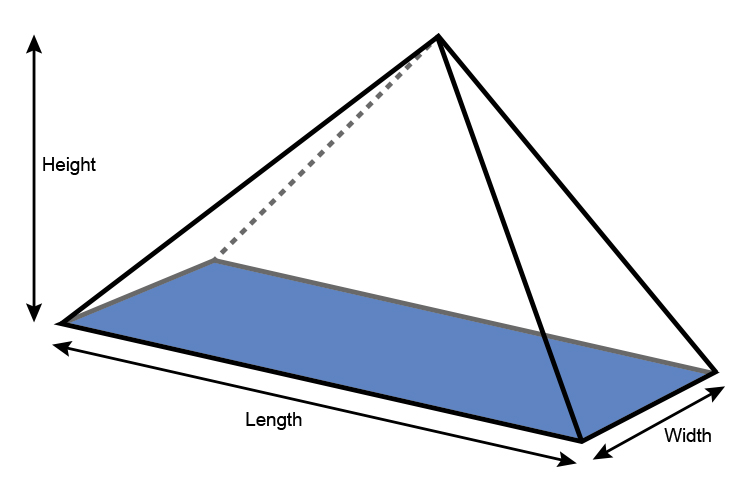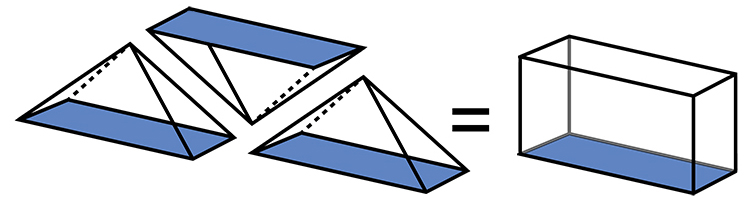# Volume of rectangular based pyramids

The volume of a rectangular based pyramid works on area of the base multiplied by height and some factor.Area of base of a rectangular based pyramid = Len\g\th\times\width

Volume of a rectangular based pyramid = 1/3times\area\ of\ base\ timesheight

Volume of a rectangular based pyramid = 1/3times\l\e\n\g\th\times\width\timesheight3 rectangular based pyramids fill 1 rectangular cuboid (with the same size base and height).

NOTE:

Areas shaded blue are all equal.